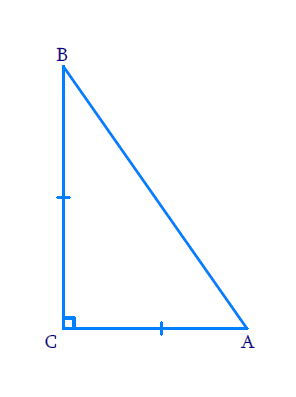# Ex.6.5 Q4 Triangles Solution - NCERT Maths Class 10

Go back to  'Ex.6.5'

## Question

$$ABC$$ is an isosceles triangle right angled at $$C.$$ Prove that $${AB}^{2}=2{AC}^{2}$$.

DiagramVideo Solution
Triangles
Ex 6.5 | Question 4

## Text Solution

Reasoning:

As we are aware, in a right triangle, the square of the hypotenuse is equal to the sum of the squares of the other two sides.

Steps:

In $$\Delta ABC,\;\angle ACB=\,90^{\circ}$$ and $$AC=BC$$

But

\begin{align} A{{B}^{2}}&=A{{C}^{2}}+B{{C}^{2}} \\ A{{B}^{2}}& =A{{C}^{2}}+A{{C}^{2}}\;\;[\because AC=BC] \\ A{{B}^{2}}&=2A{{C}^{2}} \end{align}

Learn from the best math teachers and top your exams

• Live one on one classroom and doubt clearing
• Practice worksheets in and after class for conceptual clarity
• Personalized curriculum to keep up with school